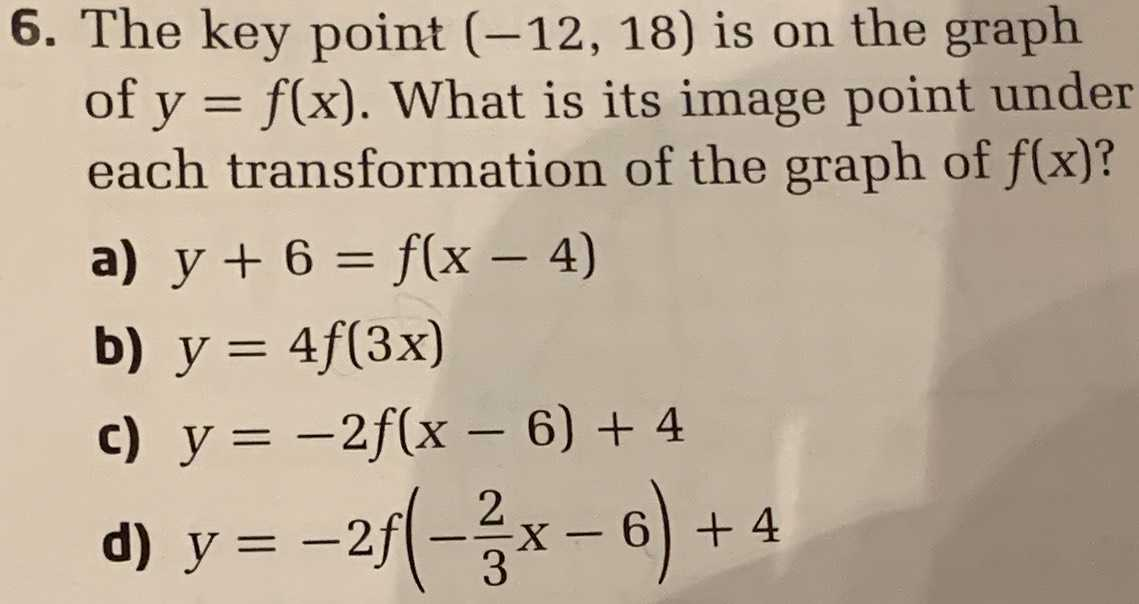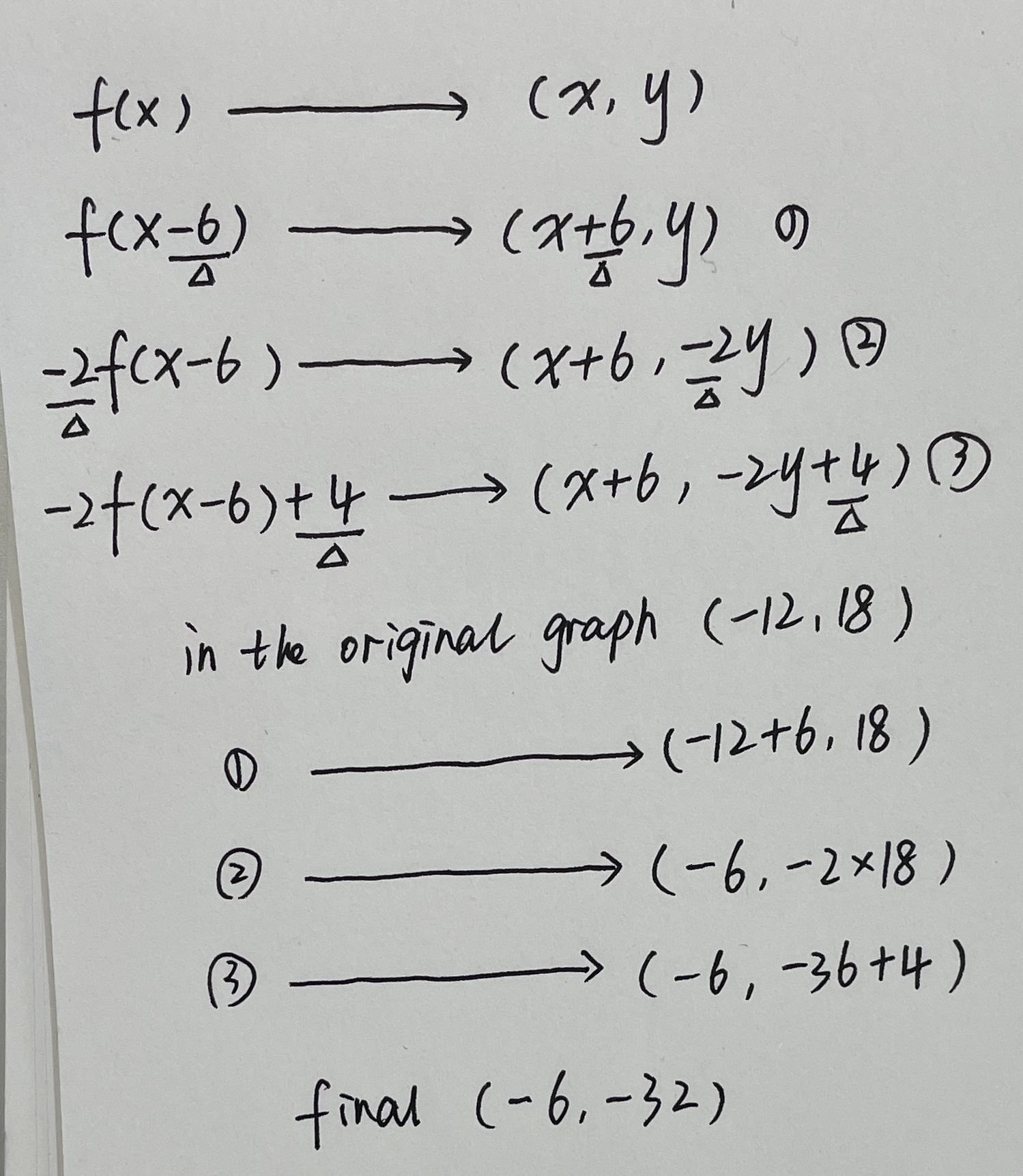### ¿Todavía tienes preguntas de matemáticas?

Pregunte a nuestros tutores expertos
Algebra
Pregunta6. The key point $$( - 12,18 )$$ is on the graph of $$y = f ( x )$$ . What is its image point under each transformation of the graph of $$f ( x )$$ ? a) $$y + 6 = f ( x - 4 )$$

b) $$y = 4 f ( 3 x )$$

c) $$y = - 2 f ( x - 6 ) + 4$$

d) $$y = - 2 f ( - \frac { 2 } { 3 } x - 6 ) + 4$$